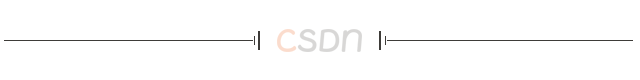# 牛客网——Java刷题篇

anan7个月前 (09-03)后端8551. 检测字符串是否为回文

2. 最后一个单词的长度

3. 第一个只出现一次的字符

# 1. 检测字符串是否为回文

1 <= s.length <= 2 * 105

``````class Solution {
public boolean isPalindrome(String s) {
// 左指针
int left = 0;
// 右指针
int right = s.length() - 1;

// 左右指针分别从前和从后往中间移动
while (left < right) {
char c1 = s.charAt(left);
char c2 = s.charAt(right);
if (!Character.isLetterOrDigit(c1)) {
// 过滤掉非字母和数字字符
left++;
continue;
}
if (!Character.isLetterOrDigit(c2)) {
// 过滤掉非字母和数字字符
right--;
continue;
}
// 忽略字母大小写
if (Character.toLowerCase(c1) != Character.toLowerCase(c2)) {
return false;
}
// 挪动指针
left++;
right--;
}
return true;
}
}``````

# 2. 最后一个单词的长度

``````import java.util.Scanner;

public class Main{
public static void main(String[] args){
//标准输入
Scanner sc=new Scanner(System.in);
//键盘输入字符串
String s=sc.nextLine();
//定义指针变量
int index=-1;
for(int i=s.length()-1;i>=0;i--){
//从后往前第一个空格的位置
if(s.charAt(i)==' '){
index=i;
break;
}
}
//总长度减去指针到开头一段的长度，即得到最后一个单词的长度
System.out.println(s.length()-index-1);
}
}
``````

``````import java.util.Scanner;

public class Main{
public static void main(String[] args){
//标准输入
Scanner sc=new Scanner(System.in);
//键盘输入字符串
String s=sc.nextLine();
//以空格分割为字符串数组
String[] arr=s.split(" ");
//字符串数组最后一个元素即是原字符串的最后一个单词，直接输出其长度
System.out.println(arr[arr.length-1].length());
}
}
``````

# 3. 第一个只出现一次的字符

• `1 <= s.length <= 105`
• `s` 只包含小写字母

1.统计各个字符出现次数

2.重新遍历字符数组

``````public int firstUniqChar(String s){
int[] count = new int;
for (int i = 0; i < s.length(); i++) {

char ch = s.charAt(i);
count[ch-'a'] ++;

}
for (int i = 0; i < s.length(); i++) {
char ch = s.charAt(i);
if(count[ch-'a'] == 1){
return i;
}
}
return -1;
}``````

“ 本期的分享就到这里了， 记得给博主一个三连哈，你的支持是我创作的最大动力！,https://blog.csdn.net/chenchenchencl/article/details/126388369### Java8新特性之Lambda表达式

Lambda目录 前言 一、Lambda表达式有哪些语法? 1.1.语法一(无参数，无返回值) 1.2.语法二(有一个参数，并且无返回值) 1....